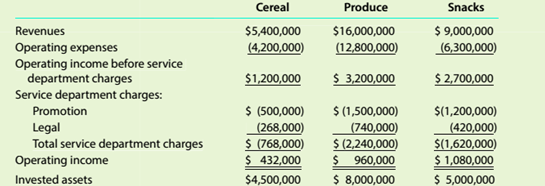Chapter 14, Problem 14.3.2C

Chapter
Section
Textbook Problem

Evaluating divisional performance The three divisions of Dixie Foods are Cereal, Produce, and Snacks. The divisions are structured as investment centers. The following responsihility reports were prepared for the three divisions for the prior yearAssuming that the minimum acceptable rate of return on new projects, is 10%. would all investments that produce a return in excess of 10% be accepted by the divisions?

To determine

Concept Introduction:

Return on investment (ROI):

Return on investment is a profitability ratio that represents the percentage return on the investment made. It is calculated by dividing the Net Income by the Average total assets. The formulas to calculate the ROI are as follows:

ROI = Operating IncomeAverage total assets

ROI (Expanded)= Operating IncomeSales ×SalesAverage total assets

Or

ROI = Profit Margin Ratio ×Asset Turnover ratio

If all the investment providing more than 10% shall be accepted

Explanation

Division which is making best use of invested assets is identified as follows:

 Cereal Produce Snacks Operating Income (A) $432,000$ 960,000 \$ 1,080,000

Still sussing out bartleby?

Check out a sample textbook solution.

See a sample solution

The Solution to Your Study Problems

Bartleby provides explanations to thousands of textbook problems written by our experts, many with advanced degrees!

Get Started

Find more solutions based on key concepts